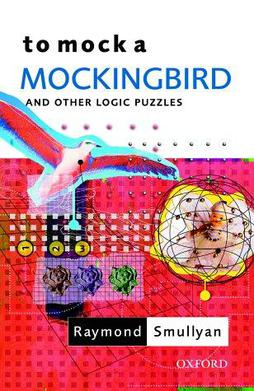### Solving a logic puzzle with the Z3 theorem proverHere's a puzzle from Raymond Smullyan's To Mock a Mockingbird:

1. The Flower Garden

In a certain flower garden, each flower was either red, yellow, or blue, and all three colors were represented. A statistician once visited the garden and made the observation that whatever three flowers you picked, at least one of them was bound to be red. A second statistician visited the garden and made the observation that whatever three flowers you picked, at least one was bound to be yellow.

Two logic students heard about this and got into an argument. The first student said: "It therefore follows that whatever three flowers you pick, at least one is bound to be blue, doesn't it?" The second student said: "Of course not!"

Which student was right, and why?

I thought it would be a nice way to test out using the Z3 theorem prover on a small puzzle. This puzzle isn't very realistic, but it's easy to see how SAT and SMT solvers can generalize to much more complicated problems where using some other kind of linear or non-linear solver would not work or would be more complicated.

First, we instantiate a new solver and define the basic variables:

In :
from z3 import *

# initialize the solver
s = Solver()

# define the integer variables for the total numbers in a realization of the problem
N_red, N_blue, N_yellow = Ints("N_red, N_blue, N_yellow")

# define the integer variables for the sampled numbers in a realization of the problem
n_red, n_blue, n_yellow = Ints("n_red, n_blue, n_yellow")

# for iteration purposes in the next step, rearrange these variables into pairs of (sampled, total)
colors = [
(n_red, N_red),
(n_blue, N_blue),
(n_yellow, N_yellow)
]


Now we add all of the hard constraints described in the puzzle:

In :
# n flowers in sample non-negative, less than N, and all colors represented in garden
for n, N in colors:
# n flowers in each sample can be from zero up to N in garden
s.add(n >= 0, n <= N)
# we are told all flowers are represented in the garden

# sample size is 3, so the sum of the samples of each color must equal 3
s.add(n_red + n_blue + n_yellow == 3)

# first statistician axiom: all samples of 3 include 1 yellow

# second statistician axiom: all samples of 3 include 1 red


With all of the hard constraints represented we will encode the conjecture in question:

In :
# first student: whatever three flowers you pick, at least one is bound to be blue
first_student_conjecture = (n_blue >= 1)

# second student: not so!
second_student_conjecture = Not(first_student_conjecture)


Finally, we add the second student's conjecture as a constraint and see if it's still possible to satisfy all the other constraints. Since the first student was making a "for all" statement, the second student can disprove it with only one "there exists" counterexample.

We add the negation of the first student's conjecture as a hard constraint and see if an example can be found that satisfies all the rest of the hard constraints:

In :
%%time
result = s.check()
if result == sat:
print("student 2 was right, counterexample:")
print(s.model())
else:
print("student 1 was right")

student 2 was right, counterexample:
[N_blue, = 1,
N_yellow = 1,
n_blue, = 0,
n_yellow = 1,
N_red, = 2,
n_red, = 2]
CPU times: user 11.1 ms, sys: 0 ns, total: 11.1 ms
Wall time: 9.34 ms


Not only could we find such a counterexample, solving was nearly instantaneous. Student 2 was right.

Note, I found Denis Yurichev's SAT/SMT guide and the Programming Z3 page helpful in understanding the basics, but it was sort of difficult to find cohesive, basic documentation out there for these kinds of solvers. Partly that is because these tools are mostly developed by research groups, but there is plenty of space for blog posts or real world case studies that others might learn from.

Any comments or suggestions? Let me know.